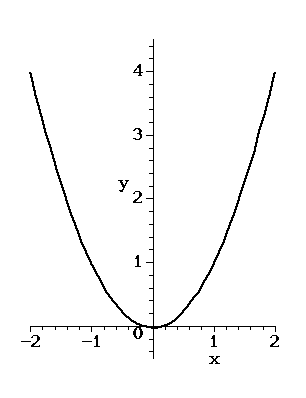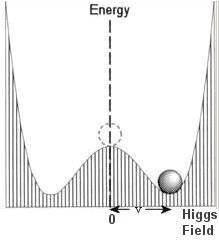# Higgs field

What is Higgs field? Some books say that because of this field the matter has the properties of mass but when I see arxiv I think it's something different. If Higgs particle is a boson then why this Higgs mechanism not considered a force?

Is anybody going to post on this thread?

malawi_glenn
Homework Helper
Maybe nobody knows why not the higgs mechanism is considered not to be a force?

I personally think that it is because Hiss field is a scalar field, not vectorial field. But I am not good at particle physics at this level... so I might be wrong.

What books have you considered?

If Higgs particle is a boson then why this Higgs mechanism not considered a force?

Hmm. This is a good question:) My first instinct is to say that it is a scalar particle, and the difference may lie there (as malawi said). I remember reading something in Tony Zee's book (QFT in a Nutshell) about a spin one particle mediates a force that is either attractive or repulsive (EM, weak, string forces), and a spin two particle mediates a force that can ONLY be attractive (gravity). I don't remember if he said anything about a spin zero particle, and I can't reconstruct the arguments in my head.

The other thing is that things aren't charged'' under the higgs field, as they are charged'' under the other forces. Electrons and quarks and such couple to the higgs field. The terms in the SM lagrangian (often called the yukawa couplings) LOOK like mass terms when the higgs gets a vacuum expectation value.

Vacuum expectation value'' just means that the field gets a value everywhere in space. There are some good pictures

Before symmetry breaking, the higgs potential looks like this:The average value is zero. Think of a pendulum oscillating back and forth---the time average of its position is zero. After symmetry breaking, the potential looks like this:The average value is now AWAY from zero.

turbo
Gold Member
Let's consider the function of the Higgs boson. It is supposed to somehow convey mass to particles. In a related particle-centered concept, we are asked to believe in the existence of graviton, that mediates the gravitational attraction between particles that have been granted the mass conferred by the Higgs boson. This particular brand of particle-based quantum cosmology is ruled out by observation. Everywhere and everywhen we look in the universe, gravitation appears to dominate on large scales and in each instance (even if galaxies and clusters don't appear to obey the gravitational rules laid down by Newton and Einstein, requiring the existence of copious amounts of dark matter to fix the shortfalls between theory and observation) gravitation appears to work in predictable ways.

Consider: If the Higgs field and the Higgs bosons and the gravitational field and gravitons are separate entities, how can the fields and the particle densities possibly have remained so incredibly congruent throughout the life-time of our visible universe? Fields are variable by definition. This is perhaps the strongest argument against the existence of the Higgs Boson (a giant sink-hole for research dollars) and the graviton, though somehow the congruence paradox has not crept into the literature.

Last edited:
Disclaimer: All mathematical expressions and the definition of the appearing symbols are to be considered sketches.

Some books say that because of this field the matter has the properties of mass but when I see arxiv I think it's something different.
There is effectively some difference between the Higgs-field (which gives masses to the particles) and the Higgs-boson (which is the waste left of the Higgs-field after masses are given).

The Higgs-field can be considered a pair of complex scalar fields. These fields are supposed to have interactions with the other fields, something like $$c \bar \psi \Phi \psi$$ with $$\psi$$ being some fermion field and $$\Phi$$ being (something like) the Higgs-field and c being the strength of the interaction. What the happens is that the state (of the world) we consider a vacuum is not the state in which $$\Phi = 0$$ but that e.g. $$\Phi = v$$. You can now define some new field which compensates this shift (and therefore is zero in the vacuum again): $$\phi := \Phi - v$$ (note that $$\Phi$$ is dynamic; v is a constant). If you substitute this new field into the original equation for the fields, the previous interaction term reads $$c \bar \psi ( \phi + v) \psi = \underbrace{c \bar \psi \phi \psi}_{I} + \underbrace{c v \bar \psi \psi}_{M}$$. The 2nd addend M is exactly what a mass term looks like in quantum field theory. In that sense, the Higgs-field is supposed to give mass to the particles (actually only for the fermions here, the terms for the gauge bosons are slightly different but it also results in that the parameter v gives them their masses). I is still an interaction term.

You now have expressed the original Higgs-field $$\Phi$$ as the offset $$\phi$$ from its value in vacuum. By a suitable redefinition of the gauge boson fields, three of the four degrees of freedom $$\phi$$ has are absorbed into the newly-defined fields (let's call them "interaction particles"). There remains one degree of freedom that is not absorbed. This degree of freedom is the Higgs-boson.

In short: There is a thing called Higgs-field. This field is redefined and partially absorbed by other fields (via their redefinition). The remaining one real-valued degree of freedom of exitation from the vacuum-state is the Higgs-boson.

If Higgs particle is a boson then why this Higgs mechanism not considered a force?
In the end, every such question boils down to "it's a matter of definition and convention". The Higgs-field is different from the fields that (after the redefinition already mentioned above) later form the particles you probably know as force carriers. The "gauge fields" (that's their name) result from demanding that there exist some abstract symmetries ("gauge symmetries" - now guess where the name "gauge boson" comes from). The Higgs-field is no such result but simply put into the Standard Model to make it work. Resulting from different approaches, the Higgs-field and the gauge fields are conceptually and practically quite different, the most obvious difference being the spin (scalar vs. vector), less obvious being that the unredefined Higgs-field must have mass terms (and even more) while the unredefined gauge fields are mathematically forbidden to have them.

In short: The Higgs-field from which the Higgs-boson stems is conceptually different from the gauge fields from which the interaction particles (photon,W,gluon,Z) originate.

Last edited: